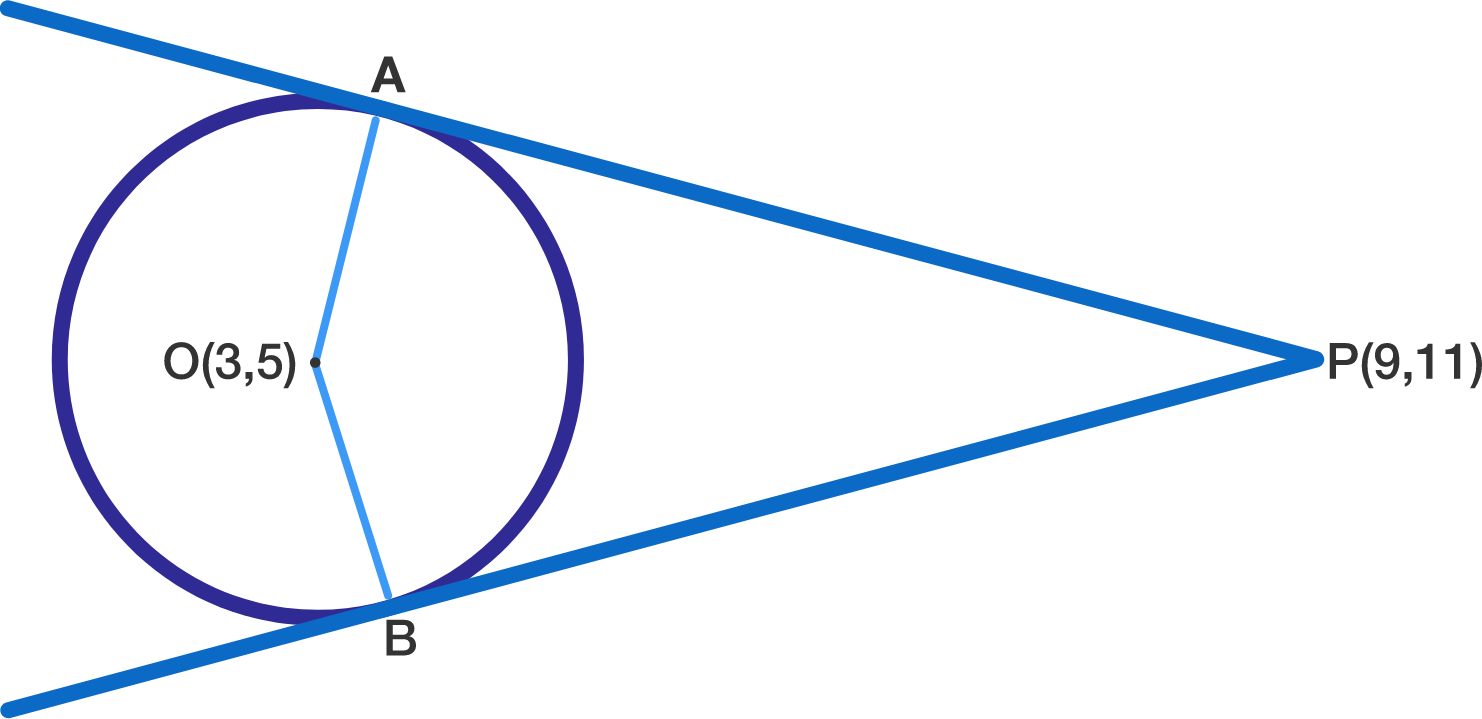# Maintaining Equal Distances

Geometry Level 3A circle with centre $O=(3,5)$ hosts a pair of tangents at $A$ and $B$ from an external point $P=(9,11)$.

Find the distance between the origin and the point inside the quadrilateral $AOBP$ which is equidistant from its four vertices.

×

Problem Loading...

Note Loading...

Set Loading...